• multiclass hinge loss定义如下： 下面的代码展示了用hinge_loss函数度量SVM分类器在二元分类问题中的使用方法： from sklearn import svm from sklearn.metrics import hinge_loss X=[,] y=[-1,1] est=svm....

Python数据分析与机器学习项目实战：http://dwz.date/ckS4转载https://www.cnblogs.com/nolonely/p/7008952.html

各种损失函数

损失函数或代价函数来度量给定的模型(一次)预测不一致的程度

损失函数的一般形式：风险函数：度量平均意义下模型预测结果的好坏损失函数分类：

Zero-one Loss，Square Loss，Hinge Loss，Logistic Loss，Log Loss或Cross-entropy Loss，hamming_loss

分类器中常用的损失函数：Zero-One Loss

该函数计算nsamples个样本上的0-1分类损失(L0-1)的和或者平均值。默认情况下，返回的是所以样本上的损失的平均损失，把参数normalize设置为False，就可以返回损失值和

在多标签分类问题中，如果预测的标签子集和真实的标签子集严格匹配，zero_one_loss函数给出得分为1，如果没有任何的误差，得分为0

from sklearn.metrics import zero_one_loss

import numpy as np

#二分类问题

y_pred=[1,2,3,4]

y_true=[2,2,3,4]

print(zero_one_loss(y_true,y_pred))

print(zero_one_loss(y_true,y_pred,normalize=False))

#多分类标签问题

print(zero_one_loss(np.array([[0,1],[1,1]]),np.ones((2,2))))

print(zero_one_loss(np.array([[0,1],[1,1]]),np.ones((2,2)),normalize=False))

#结果：

#0.25

#1

#0.5

#1

Hinge Loss

该损失函数通常被用于最大间隔分类器，比如假定类标签+1和-1，y：是真正的类标签，w是decision_function输出的预测到的决策，这样，hinge loss 的定义如下：如果标签个数多于2个，hinge_loss函数依据如下方法计算：如果y_w是对真是类标签的预测，并且y_t是对所有其他类标签的预测里边的最大值，multiclass hinge loss定义如下：下面的代码展示了用hinge_loss函数度量SVM分类器在二元分类问题中的使用方法：

from sklearn import svm

from sklearn.metrics import hinge_loss

X=[,]

y=[-1,1]

est=svm.LinearSVC(random_state=0)

print(est.fit(X,y))

pred_decision=est.decision_function([[-2],,[0.5]])

print(pred_decision)

print(hinge_loss([-1,1,1],pred_decision))

#结果

#LinearSVC(C=1.0, class_weight=None, dual=True, fit_intercept=True,

intercept_scaling=1, loss='squared_hinge', max_iter=1000,

multi_class='ovr', penalty='l2', random_state=0, tol=0.0001,

verbose=0)

#[-2.18177262 2.36361684 0.09092211]

#0.303025963688

Log Loss(对数损失)或者Cross-entropy Loss(交叉熵损失)

在二分类时，真是标签集合为{0，1}，而分类器预测得到的概率分布为p=Pr(y=1)

每一个样本的对数损失就是在给定真是样本标签的条件下，分类器的负对数思然函数，如下所示：当某个样本的真实标签y=1时，Loss=-log(p)，所以分类器的预测概率p=Pr(y=1)的概率越大，则损失越小；如果p=Pr(y=1)的概率越小，则分类损失就越大，对于真是标签y=0，Loss=-log(1-p)，所以分类器的预测概率p=Pr(y=1)的概率越大，则损失越大多分类跟这个类似，不在重复

#Log Loss

from sklearn.metrics import log_loss

y_true=[0,0,1,1]

y_pred=[[0.9,0.1],[0.8,0.2],[0.3,0.7],[0.01,0.99]]

print(log_loss(y_true,y_pred))

#0.173807336691

Hamming Loss，计算两个样本集合之间的平均汉明距离#hamming_loss

from sklearn.metrics import hamming_loss

y_pred=[1,2,3,4]

y_true=[2,2,3,4]

print(hamming_loss(y_true,y_pred))

#0.25

展开全文• sklearn常用函数的参数详解

千次阅读 2018-08-13 13:55:48
...sklearn.neighbors.KNeighborsClassifier KNneighborsClassifier参数说明： n_neighbors：默认为5，就是k-NN的k的值，选取最近的k个点。 weights：默认是uniform，参数可以是unifo...

参考资料：https://blog.csdn.net/column/details/16415.html

KNN

sklearn.neighbors.KNeighborsClassifier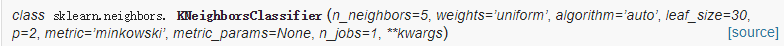KNneighborsClassifier参数说明：

• n_neighbors：默认为5，就是k-NN的k的值，选取最近的k个点。
• weights：默认是uniform，参数可以是uniform、distance，也可以是用户自己定义的函数。uniform是均等的权重，就说所有的邻近点的权重都是相等的。distance是不均等的权重，距离近的点比距离远的点的影响大。用户自定义的函数，接收距离的数组，返回一组维数相同的权重。
• algorithm：快速k近邻搜索算法，默认参数为auto，可以理解为算法自己决定合适的搜索算法。除此之外，用户也可以自己指定搜索算法ball_tree、kd_tree、brute方法进行搜索，brute是蛮力搜索，也就是线性扫描，当训练集很大时，计算非常耗时。kd_tree，构造kd树存储数据以便对其进行快速检索的树形数据结构，kd树也就是数据结构中的二叉树。以中值切分构造的树，每个结点是一个超矩形，在维数小于20时效率高。ball tree是为了克服kd树高纬失效而发明的，其构造过程是以质心C和半径r分割样本空间，每个节点是一个超球体。
• leaf_size：默认是30，这个是构造的kd树和ball树的大小。这个值的设置会影响树构建的速度和搜索速度，同样也影响着存储树所需的内存大小。需要根据问题的性质选择最优的大小。
• metric：用于距离度量，默认度量是minkowski，也就是p=2的欧氏距离(欧几里德度量)。
• p：距离度量公式。在上小结，我们使用欧氏距离公式进行距离度量。除此之外，还有其他的度量方法，例如曼哈顿距离。这个参数默认为2，也就是默认使用欧式距离公式进行距离度量。也可以设置为1，使用曼哈顿距离公式进行距离度量。
• metric_params：距离公式的其他关键参数，这个可以不管，使用默认的None即可。
• n_jobs：并行处理设置。默认为1，临近点搜索并行工作数。如果为-1，那么CPU的所有cores都用于并行工作。

KMeans

sklearn.cluster.KMeans(n_clusters=8,
init='k-means++',
n_init=10,
max_iter=300,
tol=0.0001,
precompute_distances='auto',
verbose=0,
random_state=None,
copy_x=True,
n_jobs=1,
algorithm='auto'
)

参数的意义：

• n_clusters:簇的个数，即你想聚成几类
• init: 初始簇中心的获取方法
• n_init: 获取初始簇中心的更迭次数，为了弥补初始质心的影响，算法默认会初始10次质心，实现算法，然后返回最好的结果。
• max_iter: 最大迭代次数（因为kmeans算法的实现需要迭代）
• tol: 容忍度，即kmeans运行准则收敛的条件
• precompute_distances：是否需要提前计算距离，这个参数会在空间和时间之间做权衡，如果是True 会把整个距离矩阵都放到内存中，auto 会默认在数据样本大于featurs*samples 的数量大于12e6 的时候False,False 时核心实现的方法是利用Cpython 来实现的
• verbose: 冗长模式（不太懂是啥意思，反正一般不去改默认值）
• random_state: 随机生成簇中心的状态条件。
• copy_x: 对是否修改数据的一个标记，如果True，即复制了就不会修改数据。bool 在scikit-learn 很多接口中都会有这个参数的，就是是否对输入数据继续copy 操作，以便不修改用户的输入数据。这个要理解Python 的内存机制才会比较清楚。
• n_jobs: 并行设置
• algorithm: kmeans的实现算法，有：’auto’, ‘full’, ‘elkan’, 其中 ‘full’表示用EM方式实现

LASSO回归

展开全文• xgboost调参：最佳迭代次数

千次阅读 2019-06-13 19:35:09
xgboost调参：最佳迭代次数 说明： xgboost有一个很有用的函数“cv”，这个函数可以在每一次迭代中使用交叉验证，并返回理想的决策树数量。 #coding:utf-8 from __future__ import division import sys import ...

xgboost调参：最佳迭代次数

说明：

xgboost有一个很有用的函数“cv”，这个函数可以在每一次迭代中使用交叉验证，并返回理想的决策树数量

#coding:utf-8
from __future__ import division
import sys
import pandas as pd
import xgboost as xgb
from xgboost import XGBClassifier
from sklearn import cross_validation, metrics   #Additional     scklearn functions
from sklearn.model_selection import train_test_split
from sklearn.metrics import accuracy_score,roc_auc_score, log_loss
from sklearn.grid_search import GridSearchCV   #Perforing grid search

def modelfit(alg, dtrain, predictors,useTrainCV=True, cv_folds=5, early_stopping_rounds=50):
if useTrainCV:
xgb_param = alg.get_xgb_params()
xgtrain = xgb.DMatrix(dtrain, label=predictors)
#XGBoost有一个很有用的函数“cv”，这个函数可以在每一次迭代中使用交叉验证，并返回理想的决策树数量。
cvresult = xgb.cv(xgb_param, xgtrain, num_boost_round=alg.get_params()['n_estimators'], nfold=cv_folds,
metrics='auc', early_stopping_rounds=early_stopping_rounds)
print "cvresult---", cvresult.shape
print cvresult

alg.set_params(n_estimators=cvresult.shape)
#Fit the algorithm on the data
alg.fit(dtrain, predictors, eval_metric='auc')
#Predict training set:
dtrain_predictions = alg.predict(dtrain)
dtrain_predprob = alg.predict_proba(dtrain)[:,1]

#Print model report:
print "\nModel Report"
print "Accuracy : %.4g" % metrics.accuracy_score(predictors, dtrain_predictions)
print "AUC Score (Train): %f" % metrics.roc_auc_score(predictors, dtrain_predprob)

feat_imp = pd.Series(alg.booster().get_fscore()).sort_values(ascending=False)
print "feat_imp", "*"*30
print "feature_name feature_importance_score"
print feat_imp
return cvresult.shape

if __name__ == "__main__":
# split data into X and y
X = dataset[:,0:8]
Y = dataset[:,8]

# 把数据集拆分成训练集和测试集
seed = 7
test_size = 0.33
X_train, X_test, y_train, y_test = train_test_split(X, Y, test_size=test_size, random_state=seed)
xgb1 = XGBClassifier(
learning_rate =0.1,
n_estimators=100,
max_depth=5,
min_child_weight=1,
gamma=0,
subsample=0.8,
colsample_bytree=0.8,
objective= 'binary:logistic',
scale_pos_weight=1,
seed=27)

best_n_estimators = modelfit(xgb1, X_train, y_train)
print "best_n_estimators=", best_n_estimators

展开全文• 但我在使用sklearn库中的KFold类时，如果向tqdm中传入一个KFold.split(range(100))返回之后的结果，原本美观的进度条会被替换成迭代次数的计数，如下图： 经过一番探索后搞清楚了其中的规律： 向tqdm传入的可迭代...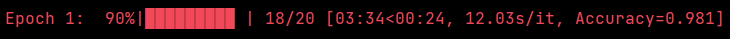如图，我们使用tqdm包中的tqdm()类时会向括号中传入一个可迭代对象，一般会显示这样一个进度条。
但我在使用sklearn库中的KFold类时，如果向tqdm中传入一个KFold.split(range(100))返回之后的结果，原本美观的进度条会被替换成迭代次数的计数，如下图：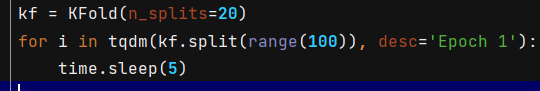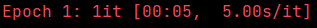经过一番探索后搞清楚了其中的规律：
向tqdm传入的可迭代对象必须被重写__len__方法，这样tqdm才能知道到底要迭代几次，因为只有这样才能计算出每次迭代所占的百分比。而KFold.split(range(100))返回的对象没有__len__方法，因此tqdm只能退而求其次数一数迭代了多少次。

解决方案：tqdm在初始化的时候有个total参数，用来显式地指定迭代的总次数。当传入的可迭代对象obj有__len__方法时，无需指定这个total参数，因为它随后会被自动地赋值为len(obj)。
在此处，我们做20折交叉验证，那么total的值显然应该等于20。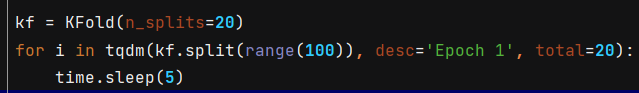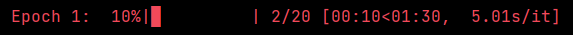展开全文• sklearn中的Logistic Regression

千次阅读 2019-04-03 12:06:59
sklearn 中，逻辑斯特回归函数来自于Logistic Regression这个类，适用于拟合0-1类，多分类（OvR），多项逻辑斯特回归（即y的值是多项的，可以是（0,1,2)），可用L1和L2正则项来优化模型。 在优化问...sklearn 逻辑斯特回归
• sklearn.KMeans使用实例 实例1 Iris,鸢尾花数据集(UC Irvine Machine Learning Repository) Iris可以从sklearn包内部导入，常常用作分类的训练数据集。这里为了方便展示聚类效果(二维在平面图中效果明显)，选取...kmeans算法 数据挖掘 python 聚类 聚类算法
• 仅在正则化优化算法为newton-cg, sag和lbfgs才有用，算法收敛的最大迭代次数。 multi_class ：分类方式选择参数，str类型，可选参数为ovr和multinomial，默认为ovr。ovr即前面提到的one-vs-rest(OvR)，而...
• 使用sklearn实现LASSO回归

千次阅读 2020-07-27 13:30:36
LASSO回归就是在正常的线性回归的基础上增加一个L1...最大迭代次数 tol 判断迭代收敛的阈值 warm_start 是否使用上一次的训练结果继续训练 属性 coef_ train_x各个特征的权重 n_iter 迭代次数 intercept_ train_x各个特机器学习
• in binary classification, the result is {}".format(logloss(y_true, y_pred)))from sklearn.metrics importlog_lossprint ("Use log_loss() in scikit-learn, the result is {}".format(log_loss(y_true, y_pred)...
• sklearn之kmeans

2020-02-25 12:00:30
sklearn.cluster.KMeans 1.参数n_clusters n_clusters是KMeans中的k，表示着我们告诉模型我们要分几类。这是KMeans当中唯一一个必填的参数，默认为8类，当我们拿到一个数据集，如果可能的话，我们希望能够通过绘图先...
• sklearn包中的K-Means算法

千次阅读 2019-06-29 22:31:56
1）函数：sklearn.cluster.KMeans 2）主要参数 n_clusters：要进行的分类的个数，即上文中k值，默认是8 ... max_iter ：最大迭代次数。默认300 min_iter ：最小迭代次数，默认10 init：有三个可选项 ...K-Means聚类
• 利用sklearn训练LDA主题模型及调参详解2017年07月31日 15:50:22阅读数：9400人生苦短，我爱python，尤爱sklearnsklearn不仅提供了机器学习基本的预处理、特征提取选择、分类聚类等模型接口，还提供了很多常用语言...
• sklearn logistic regression; 参数； 案例； 混淆矩阵。sklearn logistic 混淆矩阵
• While 簇发生变化或小于最大迭代次数： 将每个点指派到最近的质心，形成K个簇 重新计算每个簇的质心 图中有3个初始质点，形成的3个簇，再计算每个簇的质心，比较差别 # 生成数据 make_blobs import numpy as np ...
• 但在完全收敛之前，我们也可以使用max_iter，最大迭代次数，或者tol，两次迭代间Inertia下降的量，这两个参数来让迭代提前停下来。有时候，当我们的n_clusters选择不符合数据的自然分布，或者为了业务需求，必须要填...机器学习 算法 python KMeans 迭代次数
• sklearn中的KMeans算法

万次阅读 2019-08-09 20:23:20
plt.show() from sklearn.cluster import KMeans from sklearn.metrics import silhouette_samples,silhouette_score import matplotlib.pyplot as plt import matplotlib.cm as cm import numpy as np #基于轮廓...机器学习
• sklearn.cluster.KMeans(n_cluster=8,init='k-means++',n_init=10,max_iter=300,tol=0.0001, precompute_distances='auto',verbose=0,random_state=None,copy_x=True,n_jobs=1,algorithm='auto') n_cluster:类中心...
• sklearn中的聚类算法K-Means

千次阅读 2021-12-13 08:18:14
K-Means算法的平均复杂度是 O ( k ∗ n ∗ T ) O(k*n*T) O(k∗n∗T)，其中 k k k是超参数，所需要输入的簇数， n n n是整个数据集中的样本量， T T T是所需要的迭代次数（相对的，KNN的平均复杂度是 O ( n ) O(n) O...聚类 算法 sklearn
• class sklearn.gaussian_process.GaussianProcessRegressor( kernel=None, *, alpha=1e-10, optimizer='fmin_l_bfgs_b', n_restarts_optimizer=0, normalize_y=False, copy_X_train=True, random_...sklearn python 机器学习
• # -*- coding: utf-8 -*- ''' Created on 2018年1月12日 @author: Jason.F @summary: Scikit-Learn库感知器学习算法 ''' from sklearn import datasets ...from sklearn.cross_validation import train_test_s
• Sklearn之KMeans算法

万次阅读 2018-12-21 11:43:17
K-Means聚类算法的时间复杂度是O(n×k×t) ,其中n代表数据集中对象的数量，t代表着算法迭代次数，k代表着簇的数目 缺点： 1、在k-measn算法中K是事先给定的，但是K值的选定是非常难以估计的。 2、在 K-...
• from sklearn.linear_model import PassiveAggressiveClassifier >>> from sklearn.datasets import make_classification >>> >>> X, y = make_classification(n_features=4, random_...机器学习 sklearn
• Python 之 sklearn——logistic回归尽管从名字上看逻辑回归模型属于回归模型，但是实际上它是一个线性分类模型。logistic回归又称logit回归，是最大熵分类或者对数线性分类器。该模型使用逻辑函数对描述单个试验可能...
• sklearn中SVM概述及参数详解 资料一：https://www.cnblogs.com/d0main/p/10459757.html 资料二：...
• sklearn的常用函数以及参数

千次阅读 2019-09-22 19:31:30
sklearn中的算法可以分为如下几部分 分类算法 回归算法 聚类算法 降维算法 模型优化 文本预处理 其中分类算法和回归算法又叫做监督学习，聚类算法和降维算法又叫做非监督学习。 ...
• sklearn不仅提供了机器学习基本的预处理、特征提取选择、分类聚类等模型接口，还提供了很多常用语言模型的接口，LDA主题模型就是其中之一。本文除了介绍LDA模型的基本参数、调用训练以外，还将提供两种LDA调参的可行...lda代码 自然语言处理 python 机器学习 NLTK
• KMeans算法的平均复杂度是 O ( k ∗ n ∗ T ) O(k*n*T) O(k∗n∗T)，其中k是我们的超参数，所需要输入的簇数，n是整个数据集中的样本量，T是多需要的迭代次数（相对的，KNN的平均复杂度是O(n)）。在最快的情况下，...sklearn 算法 机器学习
• class sklearn.ensemble.GradientBoostingClassifier(loss='deviance', learning_rate=0.1, n_estimators=100, subsample=1.0, criterion='friedman_mse', min_samples_split=2, min_samples_leaf=1, min_...
• Sklearn的KMeans的详解

千次阅读 2018-12-26 11:10:21
这篇博文主要介绍k-means聚类算法的基本原理以及它的改进算法k-means的原理及实现步骤，同时文章给出了sklearn机器学习库中对k-means函数的使用解释和参数选择。 K-means介绍： 　K-means算法是很典型的基于距离......